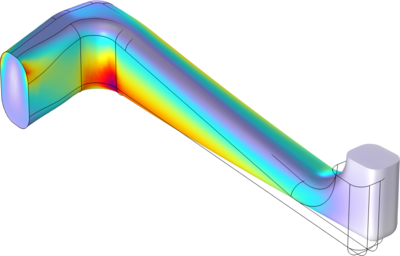Application Gallery

Static and Eigenfrequency Analyses of an Elbow Bracket

Application ID: 4131

The component depicted in this model is part of a support mechanism and is subjected to various mechanical loads. This tutorial model takes you through the steps to carry out a detailed analysis of the part using the Structural Mechanics Module.

In the various parts of the example you are introduced to using the available basic analysis types, together with numerous postprocessing possibilities. These analysis types are:

• Static analysis
• Eigenfrequency analysis
• Damped eigenfrequency analysis
• Transient analysis
• Modal-based transient analysis
• Frequency response analysis
• Modal-based frequency response analysis
• Parametric analysis
• Linear buckling analysis

This tutorial model comes in two versions:

1. A short version, elbow-bracket-brief, treating the three first analysis types in the above list.
2. A complete version, elbow-bracket, treating all nine analysis types.This model example illustrates applications of this type that would nominally be built using the following products: